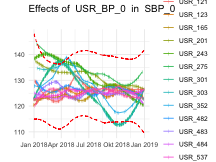# Preface

This is a brief example report using dataquieR’s functions. For a longer and better elaborated example, please also consider our online example with data from SHIP.

# INTEGRITY

## Study data

load(system.file("extdata", "study_data.RData", package = "dataquieR"))
sd1 <- study_data

The imported study data consist of:

• N = 3000 observations and
• P = 53 study variables

load(system.file("extdata", "meta_data.RData", package = "dataquieR"))
md1 <- meta_data

The imported meta data provide information for:

• P = 53 study variables and
• Q = 23 attributes

## Applicability

The call of this R-function requires two inputs only:

appmatrix <- pro_applicability_matrix(
study_data = sd1,
meta_data = md1,
label_col = LABEL
)

Heatmap-like plot:

## Segment missingness

MissSegs <- com_segment_missingness(
study_data = sd1,
meta_data = md1,
label_col = "LABEL",
threshold_value = 5,
direction = "high",
exclude_roles = c("secondary", "process")
)
MissSegs$SummaryPlot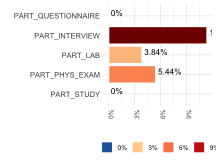### Adding variables for stratification For some analyses adding new and transformed variable to the study data is necessary. # use the month function of the lubridate package to extract month of exam date require(lubridate) # apply changes to copy of data sd2 <- sd1 # indicate first/second half year sd2$month <- month(sd2$v00013) Static metadata of the variable must be added to the respective metadata. MD_TMP <- prep_add_to_meta( VAR_NAMES = "month", DATA_TYPE = "integer", LABEL = "EXAM_MONTH", VALUE_LABELS = "1 = January | 2 = February | 3 = March | 4 = April | 5 = May | 6 = June | 7 = July | 8 = August | 9 = September | 10 = October | 11 = November | 12 = December", meta_data = md1 ) Subsequent call of the R-function may include the new variable. MissSegs <- com_segment_missingness( study_data = sd2, meta_data = MD_TMP, group_vars = "EXAM_MONTH", label_col = "LABEL", threshold_value = 1, direction = "high", exclude_roles = c("secondary", "process") ) MissSegs$SummaryPlot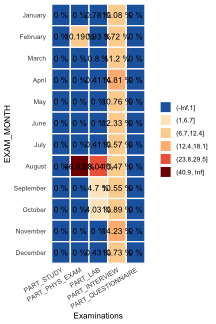## Item missingness

The following implementation considers also labeled missing codes. The use of such a table is optional but recommended. Missing code labels used in the simulated study data are loaded as follows:

code_labels <- prep_get_data_frame("meta_data_v2|missing_table")
item_miss <- com_item_missingness(
study_data = sd1,
meta_data = meta_data,
label_col = "LABEL",
show_causes = TRUE,
cause_label_df = code_labels,
include_sysmiss = TRUE,
threshold_value = 80
)

The function call above sets the analyses of causes for missing values to TRUE, includes system missings with an own code, and sets the threshold to 80%.

item_miss$SummaryTable #### Summary plot of item missingness item_miss$SummaryPlot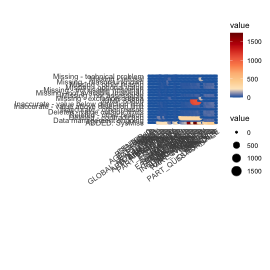# CONSISTENCY

## Limit deviations

MyValueLimits <- con_limit_deviations(
resp_vars = NULL,
label_col = "LABEL",
study_data = sd1,
meta_data = md1,
limits = "HARD_LIMITS"
)

MyValueLimits$SummaryTable ### Summary plot # select variables with deviations whichdeviate <- as.character(MyValueLimits$SummaryTable$Variables)[MyValueLimits$SummaryTable$GRADING == 1] patchwork::wrap_plots(plotlist = MyValueLimits$SummaryPlotList[whichdeviate], ncol = 2)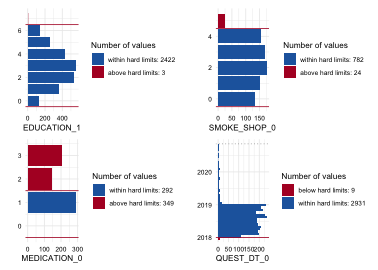IAVCatAll <- con_inadmissible_categorical(
study_data = sd1,
meta_data = md1,
label_col = "LABEL"
)

checks <- read.csv(system.file("extdata",
package = "dataquieR"
),
header = TRUE, sep = "#"
)
AnyContradictions <- con_contradictions(
study_data = sd1,
meta_data = md1,
label_col = "LABEL",
check_table = checks,
threshold_value = 1
)
AnyContradictions$SummaryTable AnyContradictions$SummaryPlot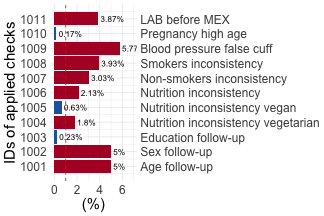# ACCURACY

ruol <- dataquieR:::acc_robust_univariate_outlier(study_data = sd1, meta_data = md1, label_col = LABEL)

c(
head(ruol$SummaryPlotList, 2), tail(ruol$SummaryPlotList, 2)
)
## $AGE_0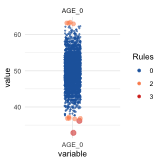## ##$AGE_1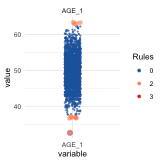##
## $ITEM_7_0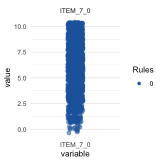## ##$ITEM_8_0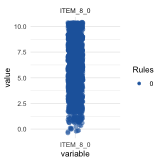myloess <- dataquieR::acc_loess(
resp_vars = "SBP_0",
group_vars = "USR_BP_0",
time_vars = "EXAM_DT_0",
label_col = "LABEL",
study_data = sd1,
meta_data = md1
)

myloess$SummaryPlotList ##$SBP_0